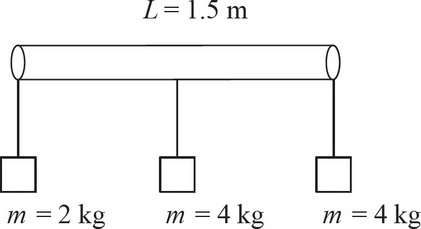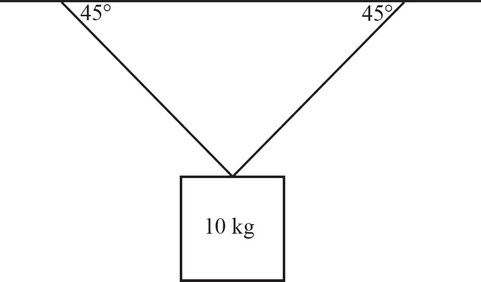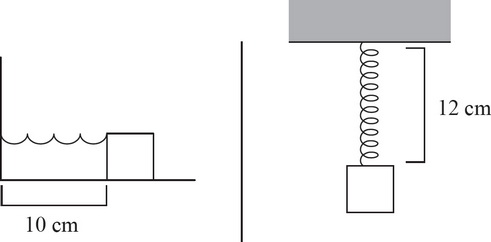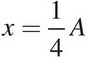# AP Physics 1 Multiple-Choice Practice Test 31

### Test Information10 questions18 minutes1. Three masses are attached to a 1.5 m long massless bar. Mass 1 is 2 kg and is attached to the far left side of the bar. Mass 2 is 4 kg and is attached to the far right side of the bar. Mass 3 is 4 kg and is attached to the middle of the bar. At what distance from the far left side of the bar can a string be attached to hold the bar up horizontally?

2. A 10 kg mass is attached to the ceiling with two strings (shown below). If the system is in static equilibrium, what is the tension in each of the strings?3. A student observes that a block oscillating on the end of a spring with an amplitude of 20 cm has a period 0.25 s. What is the period of the same oscillating block if the amplitude is increased to 60 cm ?4. A horizontal spring with a block attached to the end has a natural length of 10 cm. When the spring is attached to the ceiling, the natural length increases by 2 cm. If the spring constant of the block is 50 N/m, what is the mass of the block?

5. A simple pendulum is constructed with a 3 kg mass attached to the ceiling with a 25 cm long string. What is the magnitude of the restoring force acting on the mass when the angle to the vertical is 30° ?

6. A block attached to an ideal spring undergoes simple harmonic motion about its equilibrium position (x = 0) with amplitude A. What fraction of the total energy is in the form of potential energy when the block is at position?

7. If the distance between a crest and an adjacent trough is 0.5 m, what is the wavelength of the wave?

8. The speed of a transverse wave on a stretched string is v. If the string is replaced with one half of the linear density and the tension in the string is doubled, what is the new speed of the transverse wave?

9. What is the difference between the wavelengths of the 3rd and 4th harmonics of a standing wave fixed at both ends?

10. A speaker is projecting a sound wave through the air in the +x-direction. The energy of the sound wave is then increased. Which of the following best describes the resulting change to the wave?